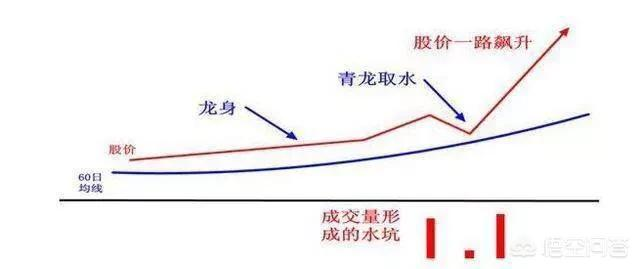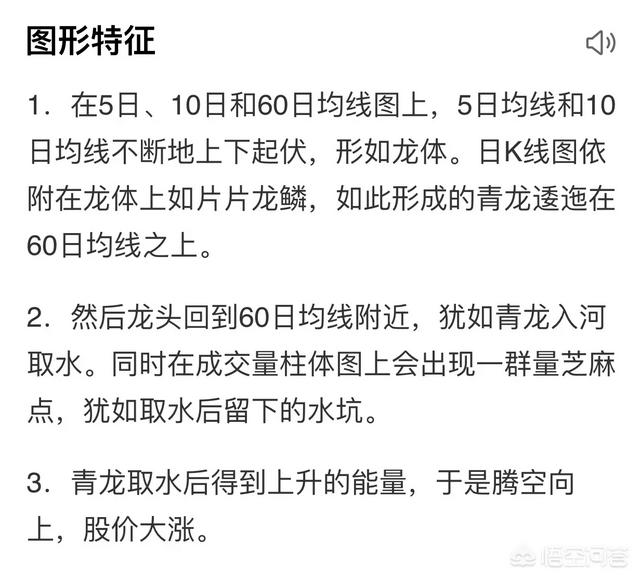# 什么是青龙取水形态，股票遇到“青龙取水”形态一定会涨吗？TJ10:=MA(C,5)>MA(C,60) AND MA(C,5)/MA(C,60)<1.10; TJ11:=MA(C,10)>MA(C,60) AND MA(C,10)/MA(C,60)<1.10; TJ1:=COUNT(TJ10 AND TJ11,90)=90; TJ2:=COUNT(MA(C,60)>REF(MA(C,60),1),90)=90; TJ3:=MIN(MA(C,5),MA(C,10))/MA(C,60)<1.05; TJ4:=COUNT(V4 AND CROSS(MA(C,5),MA(C,10));

TJ:=TJ1 AND TJ2 AND TJ3 AND TJ4;

TJ OR EXIST(TJ,9);

##### 版权声明